# Nota bene

## NB

I was looking at the J programming language yesterday and I was amused to see that it uses “NB.” to mark the rest of a line of source code as a comment, just like # in Python or // in C++. This makes comments in J look like comments in English prose.

“NB” abbreviates the Latin phrase nota bene meaning “note well.” It’s been used to mark side notes in English for about three centuries.

Most programming languages couldn’t use “NB” or “NB.” as a comment marker because it would inconsistent with conventions for identifiers, but J’s unconventional code syntax allows it to use conventional English notation for comments.

## Why J?

I was looking at J because I have a project looking at its younger sister Remora. As described in this paper,

Remora is a higher-order, rank-polymorphic array-processing programming language, in the same general class of languages as APL and J. It is intended for writing programs to be executed on parallel hardware.

J keeps the array-oriented core of APL but drops its infamous symbols. Remora syntax is even closer to the mainstream, being written like a Lisp. (Some might object that Lisp isn’t mainstream, but it sure is compared to APL or J.)

## APL comment symbol

Learning about J’s comment marker made me curious what its APL counterpart was. APL had custom symbols for everything, including comments. Comments began with ⍝ (U+235D), the idea being that the symbol looked like a lamp, giving light to the poor soul mentally parsing code.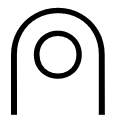The full name for the lamp symbol is “APL FUNCTIONAL SYMBOL UP SHOE JOT.” Since this section of code is explicitly for APL symbols, why not call the symbol  COMMENT or LAMP rather than UP SHOE JOT?

I suppose the comment symbol looks like the bottom of a shoe. There’s also a symbol ⍦ (U+2366)  with the name “APL FUNCTIONAL SYMBOL DOWN SHOE STILE”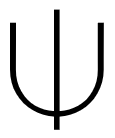and so “up” and “down” must refer to the orientation of the part of the symbol that looks like ∩ and ∪. But what about “jot” and “stile”?

A jot is a small character. The name is related to the Greek letter iota (ι) and the Hebrew letter yod (י). But if ∩ and ∪ are a shoe, the “jot” is a fairly large circle. Does “jot” have some other meaning?

A “stile” is a step or a rung, as in a turnstile. I suppose the vertical bar on top of ∪ is a stile.

## Related posts

 What is this character for in APL? Unicode includes it as an APL symbol, but it’s not included in Wikipedia’s list of APL symbols.

# Multifactorial

The factorial of an integer n is the product of the positive integers up to n.

The double factorial of an even (odd) integer n is the product of the positive even (odd) integers up to n. For example,

8!! = 8 × 6 × 4 × 2

and

9!! = 9 × 7 × 5 × 3 × 1.

Double factorials come up fairly often, and sometimes triple, quadruple, or higher multifactorials do too.

In general, the k-factorial of n is the product of the positive integers up to n that are congruent to n mod k. Here’s how you might implement k-factorials in Python.

    from operator import mul
from functools import reduce

def multifactorial(n, k):
return reduce(mul, [i for i in range(1, n+1) if (n-i)%k == 0], 1)


Update: As pointed out in the comments, multifactorial could be implemented more succinctly as

    def multifactorial(n, k):
return reduce(mul, range(n, 0, -k), 1)


Factorial notation is a little awkward, and multifactorial notation is even more awkward. Someone seeing n!! for the first time might reasonably assume it means (n!)!, but it does not.

Adding exclamation points works, albeit awkwardly, for specific values of k, but not for variable numbers of k. One way I’ve seen to express k-factorials for variable k is to add a subscript (k) to the factorial:I’ve also seen the subscript on the exclamation point written as a superscript instead.

I’d like to suggest a different notation: Πk. by analogy with the Π function.When k ≡ 1 the condition in (mod k) always holds, and we have factorial, i.e.

Π1(n) = Π(n) = n!.

One downside of this notation is that while the function Π is defined for all complex numbers (aside from singularities at negative integers) the function Πk is only defined for positive integer arguments. Still, in my opinion, this is less awkward than the alternatives I’ve seen.

By the way, the term “double factorial” seems backward. Maybe it should have been “half factorial” because you’re multiplying half as many numbers together, not twice as many. “Multifactorial” in general seems like an unfortunate name. Subfactorial might have been better, but unfortunately that name means something else.

I understand why someone may have come up with the idea of using two exclamation points for what we call double factorial; it would be hard to draw half an exclamation point. An advantage of the notation suggested here is that it suggests that there’s a variation on factorial somehow associated with k, but there’s no implication of multiplying or dividing by k.

Adding “mod k” as a subscript would be even more explicit than a subscript k. Someone who hadn’t seen

Πmod k (n)

before might be able to figure out what it means; I think they would at least easily remember what it means once told. And the presence of “mod” might suggest to the reader that the argument needs to be an integer.

# Floor, ceiling, bracket

Mathematics notation changes slowly over time, generally for the better. I can’t think of an instance that I think was a step backward.

Gauss introduced the notation [x] for the greatest integer less than or equal to x in 1808. The notation was standard until relatively recently, though some authors used the same notation to mean the integer part of x. The two definitions agree if x is positive, but not if x is negative.

Not only is there an ambiguity between the two meanings of [x], it’s not immediately obvious that there is an ambiguity since we naturally think first of positive numbers. This leads to latent errors, such as software that works fine until the first person gives something a negative input.

In 1962 Kenneth Iverson introduced the notation ⌊x⌋ (“floor of x“) and ⌈x⌉ (“ceiling of x“) in his book A Programming Language, the book that introduced APL. According to Concrete Mathematics, Iverson

found that typesetters could handle the symbols by shaving off the tops and bottoms of ‘[‘ and ‘]’.

This slight modification of the existing notation made things much clearer. The notation [x] is not mnemonic, but clearly ⌊x⌋ means to move down and ⌈x⌉ means to move up.

Before Iverson introduced his ceiling function, there wasn’t a standard notation for the smallest integer greater than or equal to x. If you did need to refer to what we now call the ceiling function, it was awkward to do so. And if there was a symmetry in some operation between rounding down and rounding up, the symmetry was obscured by asymmetric notation.

My impression is that ⌊x⌋ became more common than [x] somewhere around 1990, maybe earlier in computer science and later in mathematics.

## Iverson and APL

Iverson’s introduction of the floor and ceiling functions was brilliant. The notation is mnemonic, and it filled what in retrospect was a gaping hole. In hindsight, it’s obvious that if you have a notation for what we now call floor, you should also have a notation for what we now call ceiling.

Iverson also introduced the indicator function notation, putting a Boolean expression in brackets to denote the function that is 1 when the expression is true and 0 when the expression is false. Like his floor and ceiling notation, the indicator function notation is brilliant. I give an example of this notation in action here.

I had a small consulting project once where my main contribution was to introduce indicator function notation. That simple change in notation made it clear how to untangle a complicated calculation.

Since two of Iverson’s notations were so simple and useful, might there be more? He introduced a lot of new notations in his programming language APL, and so it makes sense to mine APL for more notations that might be useful. But at least in my experience, that hasn’t paid off.

I’ve tried to read Iverson’s lecture Notation as a Tool of Thought several times, and every time I’ve given up in frustration. Judging by which notations have been widely adopted, the consensus seems to be that the floor, ceiling, and indicator function notations were the only ones worth stealing from APL.

# Big O tilde notation

There’s a variation on Landau’s big-O notation  that’s starting to become more common, one that puts a tilde on top of the O. At first it looks like a typo, a stray diacritic mark. What does that mean? In short,That is, big O tilde notation ignores logarithmic factors. For example, the FFT computes the discrete Fourier transform of a sequence of length n in

O(n log n)

steps, and so you could write this as Õ(n). This notation comes up frequently in computational number theory where algorithms often have a mix of polynomial and logarithmic terms.

A couple days ago I blogged about algorithms for multiplying large numbers. The Schönhage-Strasse algorithm has a run time on the order of

O(n log(n) log(log(n))),

which you could write as simply Õ(n).

Shor’s quantum algorithm for factoring n-bit integers has a run time of

O(n² log(n) log(log(n))),

which we could write as Õ(n²). The fast Euclidean algorithm improves on the ancient Euclidean algorithm by reducing run time from O(n²) down to

O(n log²(n) log(log(n))),

which could be written simply as Õ(n).

The definition at the top of the post says we can ignore powers of logarithm, but the previous examples contained iterated logarithms. This is permissible because log(x) < x, and so log(log(x)) < log(x). 

## Related posts

 Big O notation can be confusing at first. For example, the equal sign doesn’t have its standard meaning. For more details, see these notes.

 Sometimes in mathematics a superscript on a function is an exponent to be applied to the output, and sometimes it indicates the number of times a function should be iterated. That is, f²(x) could mean f(x)² or f( f(x) ). The former is the convention for logarithms, and we follow that convention here.

# Misplaced decimal

This evening I ran across a dialog that suggests that decimal notation is wrong.

It happened when I started learning about decimals in school. I knew then that ten has one zero, a hundred has two, a thousand three, and so on. And then this teacher starts saying that tenth doesn’t have any zero, a hundredth has only one, a thousandth has only two, and so on. … Only much later did I have enough perspective to put my finger on the problem: The decimal point is always misplaced!

Source: Conics. Emphasis in the original.

The proposed solution is to put the decimal point above the units position rather than after it. Then the notation would be symmetric. For example, 1000 and 1/1000 would look like this: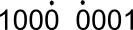Of course decimal notation isn’t likely to change, but the author makes an interesting point.

# Perpendicular and relatively prime

Donald Knuth recommends using the symbol ⊥ between two numbers to indicate that they are relatively prime. For example: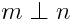The symbol is denoted \perp in TeX because it is used in geometry to denote perpendicular lines. It corresponds to Unicode character U+27C2.

I mentioned this on TeXtip yesterday and someone asked for the reason for the symbol. I’ll quote Knuth’s original announcement and explain why I believe he chose that symbol.

When gcd(m, n) = 1, the integers m and n have no prime factors in common and we way that they’re relatively prime.

This concept is so important in practice, we ought to have a special notation for it; but alas, number theorists haven’t agreed on a very good one yet. Therefore we cry: Hear us, O Mathematicians of the World! Let us not wait any longer! We can make many formulas clearer by adopting a new notation now! Let us agree to write ‘mn ’, and to say “m is prime to n,” if m and n are relatively prime.

This comes from Concrete Mathematics. In the second edition, the text is on page 115. The book explains why some notation is needed, but it does not explain why this particular symbol.

[Correction: The book does explain the motivation for the symbol. The justification is in a marginal note and I simply overlooked it. The note says “Like perpendicular lines don’t have a common direction, perpendicular numbers don’t have common factors.”]

Here’s what I believe is the reason for the symbol.

Suppose m and n are two positive integers with no factors in common. Now pick numbers at random between 1 and mn. The probability of being divisible by m and n is 1/mn, the product of the probabilities of being divisible by m and n. This says that being divisible by m and being divisible by n are independent events. Also, independent events are analogous to perpendicular lines.  The analogy is made precise in this post where I show the connection between correlation and the law of cosines.

So in summary, the ideas of being relatively prime, independent, and perpendicular are all related, and so it makes sense to use a common symbol to denote each.

# Variations on factorial!

If you’ve heard of factorial, have you heard of double factorial or subfactorial?

Double factorial is written n!!. The factorial of a positive integer n is the product of all positive integers less than or equal to n. The double factorial of n is the product of all integers less than or equal to n that have the same parity.  That is, for an odd number n,  the product defining n!! includes only odd integers and for an even integer n, the product defining n!! includes only even integers. For example, 7!! = 7 × 5 × 3 × 1 and 8!! = 8 × 6 × 4 × 2. By definition, 0!! and -1!! equal 1.

Double factorials often arise in integrals and power series and make it possible to state equations succinctly that would be verbose otherwise. For example,It’s possible to define higher factorials or multifactorials. For instance n!!!, the triple factorial of n, is the product of positive integers less than or equal to n and congruent to n mod 3. So, for example, 8!!! = 8 × 5 × 2.

Factorials count the number of ways a set can be arranged. A set with n distinct elements can be arranged in n! ways. The number of arrangements that move every element from its original position is the subfactorial of n. Sometimes subfactorial is written with the exclamation point in front of its argument and sometimes it is written with an inverted exclamation point following its argument, i.e.(By the way, the inverted exclamation mark, used to mark the beginning of an exclamatory sentence in Spanish, is Unicode character U+00A1. You can produce it in HTML with &iexl;. In TeX, you can produce it ! outside of math mode and mbox{!} in math mode.)

Subfactorial can be computed from the factorial by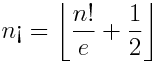for positive n where ⌊x⌋ is the greatest integer less than x. The subfactorial of 0 is defined to be 1.

Update: See post on mutifactorials.

# Four uncommon but handy math notations

Here are some of my favorite notations that are not commonly used.

The first is Richard Stanley’s notation for counting the number of ways to select k objects from a set of n objects with replacement. This is similar to the problem solved by binomial coefficients, but not the same since binomial coefficients count the number of possible selections without replacement. Stanley’s symbol is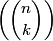I like this symbol for two reasons. First, it’s good to have a notation, any notation, for a concept that comes up fairly often. Second, it’s appropriate for this symbol to resemble the binary coefficient symbol. See selecting with replacement for more on Stanley’s symbol, how to think about it and how to compute it.

Next is Kenneth Iverson’s notation for indicator functions. Iverson’s idea was to put a Boolean condition in square brackets to indicate the function that is 1 when that condition is true and 0 otherwise. For example, [x > y] is the function f(x, y) such that f equals 1 when x is greater than y and equals 0 for all other arguments. This notation saves ink and makes it easier to concentrate on the substance of an expression. For more on Iverson’s notation, see Concrete Mathematics.

Another notation from Concrete Mathematics is the use of a perpendicular symbol to note that two integers are relatively prime. For example, mn would indicate that that m and n are relatively prime. The more common way to denote this would be to say gcd(m, n) = 1. The perpendicular symbol is nice because perpendicular lines have no component of direction in common, just as relative prime numbers have no prime factors in common.

Finally, multi-index notation is a handy way to make multivariable theorems easier to remember. For example, with this notation, Taylor series in several variables look similar to Taylor series in one variable.